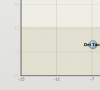Recommended Posts

Hi there,

I am creating a bubble chart with fusionchart-xt and the xml format.  I have problems with the vertical scale.  I want the horizontal lines to have an opacity of 0.15 and the labels of 1.  I cannot succeed to do it.  In short, I want the vertical scale similar to the horizontal one in the attach image.

I attach an xml file and image.

cheers!

didier

bubble.xmlShare on other sites

Hi,

As per your shared XML data, this JSFiddle is created : http://jsfiddle.net/vishalika/58sYu/377/

Can you check and point out the requirement in this?

Awaiting response.

Share on other sites

Thanks Vishalika,

I did not succeed to save the fiddle but you will find bellow the xml that generated the pale y axis.

cheers!

didier

<graph exportEnabled="0"  showYAxisValues="0" divLineAlpha="0" showLimits="0" yAxisMinValue="-9.0" xAxisMinValue="-0.110731989318" showAboutMenuItem="0" yAxisMaxValue="32.0" showAlternateHGridColor="0" canvasPadding="0" showZeroPlane="0" showToolTip="1" showVLimits="0" xAxisMaxValue="0.582691758319" yAxisName="Value"  bubbleScale="0.7" subcaption="Latest data is from 14/09/2015">
<categories>
<category showVerticalLine="1" x="-0.110731989318" label="-0.1"/>
<category showVerticalLine="1" x="-0.010731989318" label="-0.0"/>
<category showVerticalLine="1" x="0.089268010682" label="0.1"/>
<category showVerticalLine="1" x="0.189268010682" label="0.2"/>
<category showVerticalLine="1" x="0.289268010682" label="0.3"/>
<category showVerticalLine="1" x="0.389268010682" label="0.4"/>
<category showVerticalLine="1" x="0.489268010682" label="0.5"/>
<category showVerticalLine="1" x="0.582691758319" label="0.6"/>
</categories>
<dataset showValues="1">
<set name="sdsdsd" color=""
x="0.3"
y="25.5"
z="28.1"
tooltext="dsssd: X.1"
/>
<set name="sdsdsd" color=""
x="0.3"
y="0.4"
z="5.0"
tooltext="0"
/>
<set name="sddssd" color="FF5555"
x="-0.0"
y="-2.5"
z="0.9"
tooltext="sddsds.9"
/>
<set name="sdjdsd" color=""
x="0.5"
y="3.7"
z="7.2"
tooltext="2"
/>
</dataset>
<trendlines>
<line alpha="15" color="948A54" displayValue=" " endValue="2.05934018754" isTrendZone="1" startValue="-9.0"/>
<line alpha="15" startValue="-9.0" displayValue="-9"/>
<line alpha="15" startValue="-3.0" displayValue="-3"/>
<line alpha="15" startValue="3.0" displayValue="3"/>
<line alpha="15" startValue="9.0" displayValue="9"/>
<line alpha="15" startValue="15.0" displayValue="15"/>
<line alpha="15" startValue="21.0" displayValue="21"/>
<line alpha="15" startValue="27.0" displayValue="27"/>
<line alpha="15" startValue="32.0" displayValue="32"/>
</trendlines>
<vtrendlines>
<line alpha="15" color="948A54" displayValue=" " endValue="0.310206573411" isTrendZone="1" startValue="-0.11"/>
</vtrendlines>
<styles>
<definition>
<style size="10" color="000000" bold="1" name="boldfont" type="font" />
<style size="10" color="555555" bold="1" name="subcaptionfont" type="font" />
<style size="10" color="000000" bold="0" name="defaultfont" type="font" />
</definition>
<application>
<apply toObject="CAPTION" styles="boldfont" />
<apply toObject="SUBCAPTION" styles="subcaptionfont" />
<apply toObject="XAXISNAME" styles="defaultfont" />
<apply toObject="YAXISNAME" styles="defaultfont" />
<apply toObject="DATAVALUES" styles="boldfont" />
</application>
</styles>
</graph>

Share on other sites

Hi Vishalika,

Thanks a lot for your help.  On the fiddle you shared, all the axis are light, I need them darker.  Only the axis labeld ( -9, -3, 3 , ...) not the grid itself.  Can we do that with fusion charts?

thanks

didier

Share on other sites

Hi,

Try setting the alpha value in the category element of the JSFiddle shared.

Hope this helps.

Create an account or sign in to comment

You need to be a member in order to leave a comment

Create an account

Sign up for a new account in our community. It's easy!

Register a new account